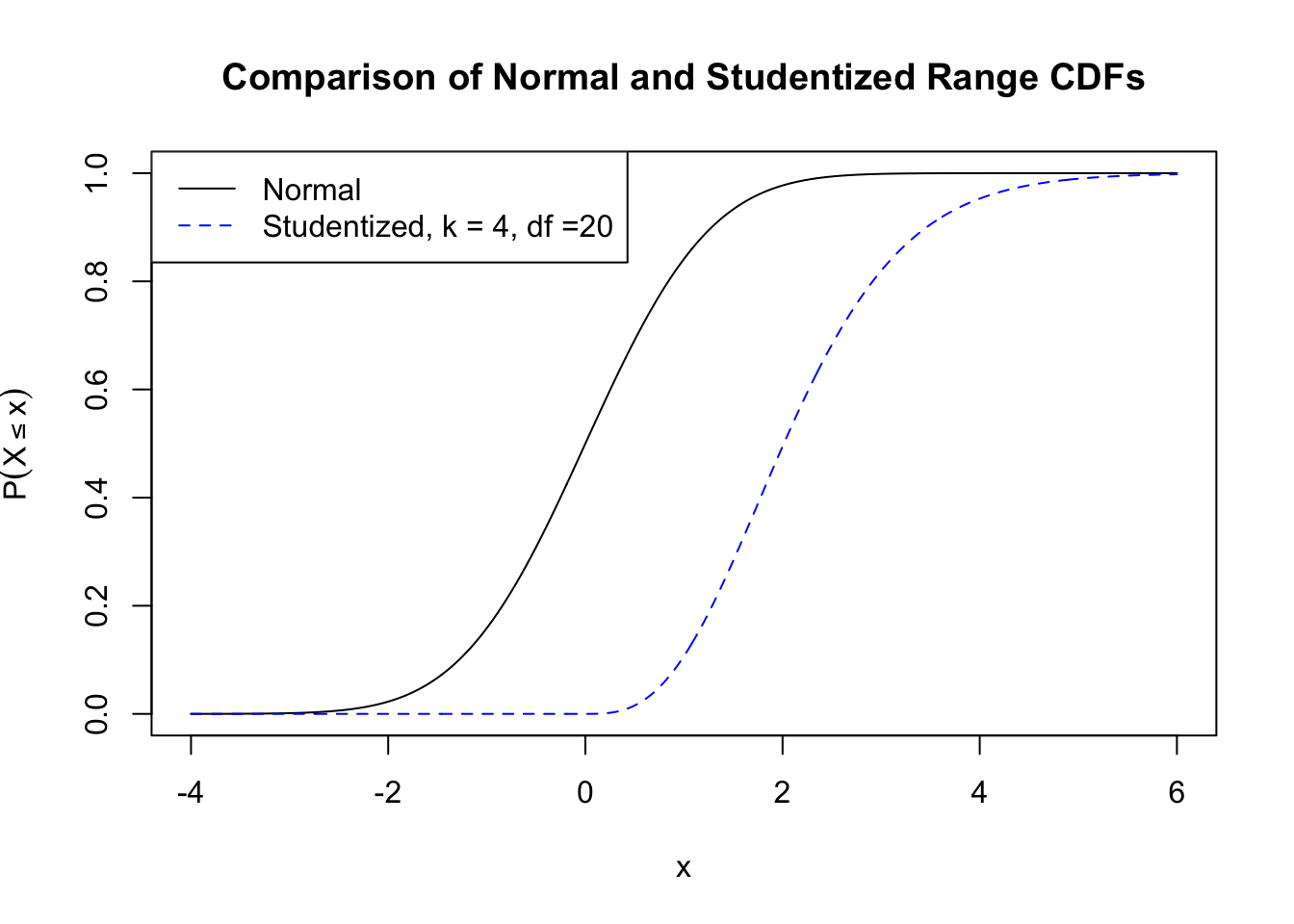# Class Slides and Documents

Class slides and documents will be posted here.

## Week 1

### Sept. 5

Class slides

Class slides - in class

## Week 2

### Sept. 10

Class slides

Class slides - inclass

### Sept. 12

Class slides

Class slides - in class

## Week 3

### Sept. 17

Class slides

Class slides - inclass

### Sept. 19

Class slides

Class slides - inclass

Live coding demo from class examined each line from the R code below.

library(BHH2)
data(shoes.data)
shoes.data

diff <- shoes.data$matA-shoes.data$matB

N <- 2^(10) # number of treatment assignments
res <- numeric(N) #vector to store results
LR <- list(c(-1,1)) # difference is multiplied by -1 or 1 # generate all possible treatment assign
trtassign <- expand.grid(rep(LR, 10))
for (i in 1:N) {
res[i] <- mean(as.numeric(trtassign[i,])*diff) }
trtassign[1:2,]

trtassign
dim(trtassign)

meandiff <- mean(diff)

res <= meandiff

sum(res <= meandiff)

## Week 4

### Sept. 24

Class slides

Class slides -inclass

### Sept. 26

Class slides

Class slides -inclass

## Week 5

### Oct. 1

Class slides

Class slides - inclass

Midterm test #1

## Week 7

### Oct. 15

Class slides

Class slides -inclass

### Oct. 17

Class slides

Class slides - inclass

The data used in class from NHEFS Survey is available nhefshw2dat.csv. The R code to read the data into an R data frame, and create the covariates used in the analyses done in class is below.

# Read the data into a data frame
#
nhefshwdat <- read.csv("nhefshw2dat.csv")

# Define the variables used in the propensity score model
#
years1 <- mean(nhefshwdat$age[nhefshwdat$qsmk == 1])
years0 <- mean(nhefshwdat$age[nhefshwdat$qsmk == 0])
male1 <- 100 * mean(nhefshwdat$sex[nhefshwdat$qsmk == 1] == 0)
male0 <- 100 * mean(nhefshwdat$sex[nhefshwdat$qsmk == 0] == 0)
white1 <- 100 * mean(nhefshwdat$race[nhefshwdat$qsmk == 1] == 0)
white0 <- 100 * mean(nhefshwdat$race[nhefshwdat$qsmk == 0] == 0)
university1 <- 100 * mean(nhefshwdat$education.code[nhefshwdat$qsmk == 1] == 5)
university0 <- 100 * mean(nhefshwdat$education.code[nhefshwdat$qsmk == 0] == 5)
kg1 <- mean(nhefshwdat$wt71[nhefshwdat$qsmk == 1])
kg0 <- mean(nhefshwdat$wt71[nhefshwdat$qsmk == 0])
cigs1 <- mean(nhefshwdat$smokeintensity[nhefshwdat$qsmk == 1])
cigs0 <- mean(nhefshwdat$smokeintensity[nhefshwdat$qsmk == 0])
smoke1 <- mean(nhefshwdat$smokeyrs[nhefshwdat$qsmk == 1])
smoke0 <- mean(nhefshwdat$smokeyrs[nhefshwdat$qsmk == 0])
noexer1 <- 100 * mean(nhefshwdat$exercise[nhefshwdat$qsmk == 1] == 2)
noexer0 <- 100 * mean(nhefshwdat$exercise[nhefshwdat$qsmk == 0] == 2)
inactive1 <- 100 * mean(nhefshwdat$active[nhefshwdat$qsmk == 1] == 2)
inactive0 <- 100 * mean(nhefshwdat$active[nhefshwdat$qsmk == 0] == 2)

## Week 8

### Oct. 22

Class slides

Class slides -inclass

Data from the blood coagualtion study is here, and can be read into an R data frame using the floowing code:

tab0401 <- read.table("tab0401.dat", header=TRUE, quote="\"")
head(tab0401) # print first 6 rows of data frame
##   run diets  y
## 1  20     A 62
## 2   2     A 60
## 3  11     A 63
## 4  10     A 59
## 5   5     A 63
## 6  24     A 59

### Oct. 24

Class slides

Class slides - inclass

In class problem

## Week 9

### Oct. 29

Class slides

Class slides - inclass

### Oct. 31

Class slides

Data files: pilotplant.xlsx; tab0502.dat; tab0503.dat

Class slides - inclass

## Week 10

### Nov. 12

Class slides

Class slides -inclass

## Week 11

### Nov. 21

Class slides

Class slides - inclass

A plot of the $$N(0,1)$$ and Studentized range (comparing k=4 means with df=20) CDFs.

p <- seq(-4, 6, by =0.01) # generate a sequence of points

plot(p, pnorm(p), type = "l", ylab = expression(P(X<= x)),
xlab = "x", col= "black",lty = 1,
main = "Comparison of Normal and Studentized Range CDFs"); # plot normal CDF

# add Studentized range cdf
points(p, ptukey(p,nmeans = 4, df = 20, lower.tail = T), col = "blue", type = "l", lty = 2);

# add plot legend
legend("topleft", col = c("black","blue"), lty = 1:2,
legend = c("Normal","Studentized, k = 4, df =20"))## Week 12

### Nov. 26

Class slides

Class slides -inclass

### Nov. 28

Class slides

Class slides -inclass

## Week 13

### Dec. 3

Class slides

Class slides -inclass

### Calculating Standard Errors in $$2^k$$ Factorial Designs

Consider the data from a replicated $$2^3$$ design from class 14.

run T C K y
6 -1 -1 -1 59
2 1 -1 -1 74
1 -1 1 -1 50
5 1 1 -1 69
8 -1 -1 1 50
9 1 -1 1 81
3 -1 1 1 46
7 1 1 1 79
13 -1 -1 -1 61
4 1 -1 -1 70
16 -1 1 -1 58
10 1 1 -1 67
12 -1 -1 1 54
14 1 -1 1 85
11 -1 1 1 44
15 1 1 1 81

The factorial effects for T C K are twice the regression coefficients: $$2\hat \beta_i$$. So, the standard error of the factorial estimates are

$s.e.(2\hat \beta_i)=\sqrt {Var(2\hat\beta_i)}=\sqrt {4Var(\hat\beta_i)}=2\sqrt{Var(\hat\beta_i)}=2s.e.(\hat \beta_i)$

fact.mod <- lm(y ~ T*C*K, data = tab0503)
summary(fact.mod)
##
## Call:
## lm(formula = y ~ T * C * K, data = tab0503)
##
## Residuals:
##    Min     1Q Median     3Q    Max
##  -4.00  -1.25   0.00   1.25   4.00
##
## Coefficients:
##               Estimate Std. Error t value Pr(>|t|)
## (Intercept)  6.425e+01  7.071e-01  90.863 2.40e-13 ***
## T            1.150e+01  7.071e-01  16.263 2.06e-07 ***
## C           -2.500e+00  7.071e-01  -3.536 0.007670 **
## K            7.500e-01  7.071e-01   1.061 0.319813
## T:C          7.500e-01  7.071e-01   1.061 0.319813
## T:K          5.000e+00  7.071e-01   7.071 0.000105 ***
## C:K          7.216e-16  7.071e-01   0.000 1.000000
## T:C:K        2.500e-01  7.071e-01   0.354 0.732810
## ---
## Signif. codes:  0 '***' 0.001 '**' 0.01 '*' 0.05 '.' 0.1 ' ' 1
##
## Residual standard error: 2.828 on 8 degrees of freedom
## Multiple R-squared:  0.9763, Adjusted R-squared:  0.9555
## F-statistic: 47.05 on 7 and 8 DF,  p-value: 7.071e-06

For example, the factorial estimate for T is $$2\times 11.5 = 23$$, and the standard error of the factorial estimates are: $$2 \times .7071=1.412$$. Confidence intervals for the factorial estiamtes are:

2*confint(fact.mod)
##                  2.5 %     97.5 %
## (Intercept) 125.238818 131.761182
## T            19.738818  26.261182
## C            -8.261182  -1.738818
## K            -1.761182   4.761182
## T:C          -1.761182   4.761182
## T:K           6.738818  13.261182
## C:K          -3.261182   3.261182
## T:C:K        -2.761182   3.761182This work is licensed under a Creative Commons Attribution-NonCommercial-ShareAlike 4.0 International License.